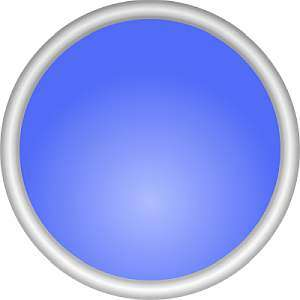# Circumference to Diameter Calculator

Instructions: Use this calculator to compute the diameter of a circle from its circumference, showing all the steps. Please type in the value of the circumference in the form below.Enter the circumference. It must a positive numeric expression (Ex: 2, or 2/3, etc.)

Passing from circumference to diameter is something that is often needed, and this calculator will allow you to do so. All you need to provide is a valid numeric expression such as '1/3' or '4', etc. The only restriction is that the expression provided needs to be positive.

Once you provide a valid diameter (it has to be positive numeric expression), you need to click on the "Calculate" button, and you will be provided with the calculations and all the steps.

This calculator is tightly related to the calculator that takes the diameter to circumference, only it is the reverse process.## How to go from circumference to diameter?

The key of the process is to use the basic formula that links circumference and diameter. We have the following formula:

$C = \pi d$

This is, the circumference corresponds to multiplying π by d. Now solving for d, we find directly that:

$d = \displaystyle \frac{C}{\pi}$

Then, to go from circumference to diameter, you just divide the circumference by π.

## What are the steps to go from circumference to diameter?

• Step 1: Identify the circumference and its potential length unit. It needs to be positive otherwise you cannot continue
• Step 2: Once you have a valid circumference C, you divide it by π to get the diameter
• Step 3: The diameter preserves the same length unit as the circumference, if any is provided.
• Step 4: The diameter may be expressed in terms of π. You may leave it as it is, or get its approximate numerical value using an expression calculator.

It is customary to leave the results in terms of π, simplifying as much as possible. Sometimes you will want to have an idea of the numerical value, in which case, it is fine to use a calculator to do that.

## How many diameter is a circumference?

The circumference is exactly π diameters. That is the magic of the constant π, which provides the link between circumference and diameter.

In a certain way, π captures the way of how there is not rational relationship between straight lengths and circular lengths.

## Why would care about computing the diameter from the circumference?

It is an option to be given either the area or the circumference of a circle, in which case, it could be useful to be able to obtain the diameter from it, or for the sake of the the same purpose, to know the radius.### Example: Calculating diameter from the circumference

Calculate the diameter, if the circumference is known to be \$$3\\pi\$$

Solution: We need to find the diameter $$d$$ of the circle, and from the information provided, we know that the circumference of the circle is $$C = 3\pi$$.

Now, the formula for the circumference is $$C = 2\pi r$$, but since the diameter is equal to twice the perimeter, we have that $$d = 2r$$, and hence, the circumference formula becomes:

$C = d \pi$

The above formula, shows how to express the circumference in terms of the diameter, and we can also solve the formula for $$d$$:

$d = \displaystyle\frac{C}{\pi}$

Therefore, all we need to do is to plug into the above formula the known value of the circumference $$C = 3\pi$$. The following is obtained:

$\begin{array}{ccl}\displaystyle d & = & \displaystyle\frac{C}{\pi} \\\\ \\\\ & = & \displaystyle\frac{3\pi}{\pi} \\\\ \\\\ & = & \displaystyle 3 \end{array}$

This concludes the calculation. We have found that the diameter of the circle is $$\displaystyle d = 3$$.

### Example: Circumference to diameter

If know that the circumference of a circle is $$4\pi$$, what is its diameter?

Solution: We need to find the diameter $$d$$ of the circle, and in this case we know that the circumference of the circle is $$C = 4\pi$$.

We need to use the formula:

$d = \displaystyle\frac{C}{\pi} = \displaystyle\frac{4\pi}{\pi} = 4$

Hence, the diameter is $$\displaystyle d = 4$$.

### Example: Another circumference to diameter

Assume that half the circumference is $$\frac{3\pi}{2}$$. Find the diameter of the circle.

Solution: In this case, we are not provided with the circumference, but with half of the circumference instead, which is $$\frac{3\pi}{2}$$ .

Hence, the circumference is $$C = 2 \cdot \frac{3\pi}{2} = 3\pi$$. So then, now we can use the formula:

$d = \displaystyle\frac{C}{\pi} = \displaystyle\frac{3\pi}{\pi} = 3$

Hence, the diameter is $$\displaystyle d = 3$$.

## More circle calculators

You will find circles wherever you go in Math. You will need to compute the area of the circle, the circumference of the circle, you name it .

Also, when dealing with circles you will want to do angle conversions, such as radians to degrees or degrees to radians.